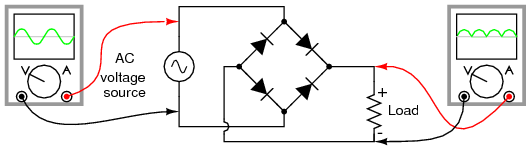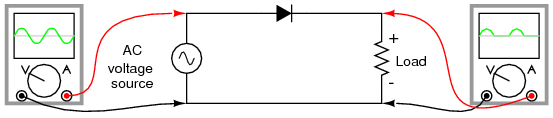# DC to the Arduino ?

Hello everyone,

i have a question that the Direct Current is in sine wave shape, if the frequency of DC can be found by the Arduino ?

i wanna use the frequency to determine the speed of motor

Thanks

If the voltage is in "sine wave shape" then it is NOT "Direct Current" (DC), it is Alternating Current (AC).

Assuming you can process the signal into some train of pulses which an Arduino should be able to count. There should be examples of code to have the Arduino count pulses (or perform the frequency-counting or tachometer) function.

This Hardware Interfacing forum would be the right place to ask questions about how to get a good countable signal from your circuit.

The Software Interfacing forum would be the right place to ask about tachometer or frequency-counting or pulse-counting routines.

Thanks
i misunderstanded the sine wave shape, sorry
i meant that the DC is in hard wave, right?
when i interfaced the motor with 4 diodes, as the figure shown below,
i could not see the frequency in the oscillator.
Can the frequency be found by the Arduino?
i am not so sure about the function inside the Arduino.i meant that the DC is in hard wave, right?

no you should have said:-
i meant that the DC is in half wave, right?

However you have got full wave and that doubles the frequency.

i could not see the frequency in the oscillator.

I assume you mean the oscilloscope! Are you turning the motor by hand and expecting it to be a generator or is it a source of power. Either way you should see a signal, if you do not you are doing something wrong.

You need some sort of way to turn this signal into a digital signal if you want to measure the frequency. How much voltage have you here? A simple transistor should be enough to square up the signal and then you can measure it by either using the pulseIn() function (for both high and low periods) or counting how many pulses you get in a specific period of time.

ouch it is typo mistake
thanks mike

yea, it is oscilloscope :-[

There is a 12V battery powering the motor, and the sensor output is around 10V and AC, and the frequency in AC is about 3.2kHz

i would like to convert it to DC, half-wave should be like this?If i have half wave, it won't be double frequency, right ?

And the transistor you mentioned, it can help me to change the DC half-wave into square wave ?

If i have half wave, it won't be double frequency, right ?

Correct

And the transistor you mentioned, it can help me to change the DC half-wave into square wave ?

Yes 10K resistor to the +ve of the load, other end of resistor into base. Emitter to ground of arduino and ground of external source. Collector to 10K and a digital input of the arduino, other end of the 10K to +5V.

Now you need to add one more resistor, this depends on the peak voltage output of the AC source (not RMS or peak to peak). It should be placed between the base and ground. It's value should be such that when it forms a potential divider with the 10K at half the peak voltage there is 0.7V across it. So for a 12V peak AC voltage source you will have a 1K3 resistor from base to ground. You won't get this value so a 1K2 will do fine.

However the frequency you will see will be the frequency of the AC voltage.

So it sounds like you are powering a motor from DC, and you have some ac "ripple" on the line which correlates with the motor speed. How big is the AC signal? (how many millivolts, etc.)

I would AC-couple from the motor to extract just the AC part, and then process it through a comparator like an LM382. That will turn the AC ripple signal into a nice hard digital pulse train which can be counted by an Arduino (running a frequency counter or tachometer sketch) The LM392 also contains an op-amp which could be used to amplify the AC signal if it is too weak to reliably detect.

Thanks Richard
but it sounds like difficult, for me.

Yes 10K resistor to the +ve of the load, other end of resistor into base. Emitter to ground of arduino and ground of external source. Collector to 10K and a digital input of the arduino, other end of the 10K to +5V.

umm..
Diodes are still needed for conversion, right ?
Is it the same output if i put the diodes in the front of transistor or back of the transistor?

Diodes are still needed for conversion, right

Yes the transistor circuit description was from the load towards the arduino, you still need the diode (for half wave) or four diodes (for full wave) from the AC power source.

thanks Mike